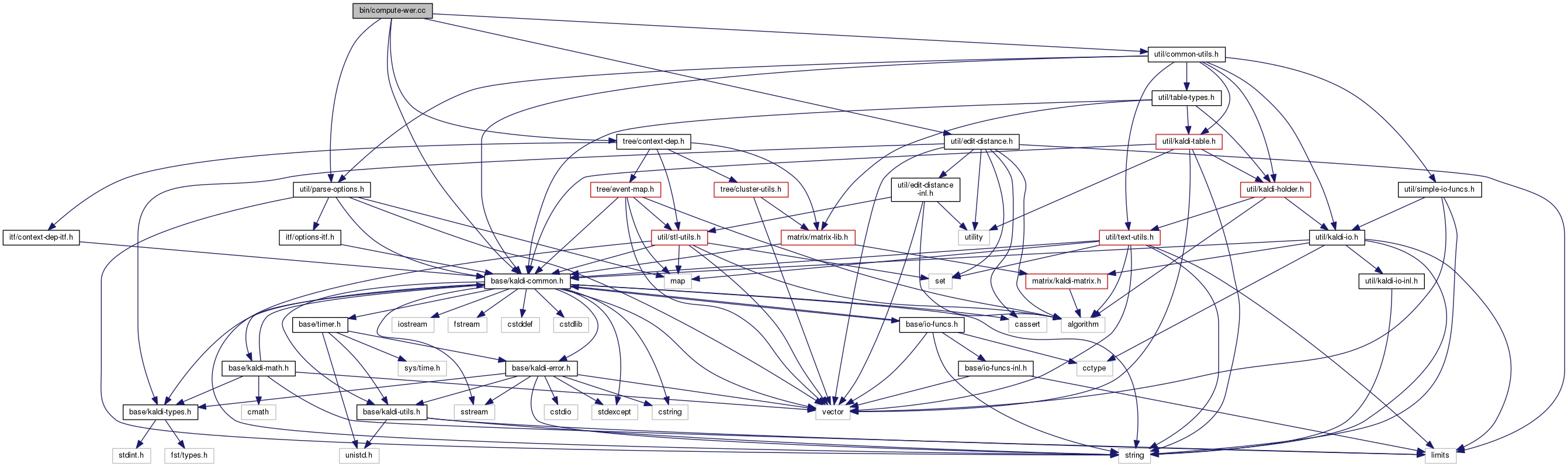compute-wer.cc File Reference
`#include "base/kaldi-common.h"`
`#include "util/common-utils.h"`
`#include "util/parse-options.h"`
`#include "tree/context-dep.h"`
`#include "util/edit-distance.h"`
Include dependency graph for compute-wer.cc:Go to the source code of this file.

## Functions

int main (int argc, char *argv[])

## ◆ main()

 int main ( int argc, char * argv[] )

Definition at line 28 of file compute-wer.cc.

28  {
29  using namespace kaldi;
30  typedef kaldi::int32 int32;
31
32  try {
33  const char *usage =
34  "Compute WER by comparing different transcriptions\n"
35  "Takes two transcription files, in integer or text format,\n"
36  "and outputs overall WER statistics to standard output.\n"
37  "\n"
38  "Usage: compute-wer [options] <ref-rspecifier> <hyp-rspecifier>\n"
39  "E.g.: compute-wer --text --mode=present ark:data/train/text ark:hyp_text\n"
41  "Example scoring script: egs/wsj/s5/steps/score_kaldi.sh\n";
42
43  ParseOptions po(usage);
44
45  std::string mode = "strict";
46  po.Register("mode", &mode,
47  "Scoring mode: \"present\"|\"all\"|\"strict\":\n"
48  " \"present\" means score those we have transcriptions for\n"
49  " \"all\" means treat absent transcriptions as empty\n"
50  " \"strict\" means die if all in ref not also in hyp");
51
52  bool dummy = false;
53  po.Register("text", &dummy, "Deprecated option! Keeping for compatibility reasons.");
54
56
57  if (po.NumArgs() != 2) {
58  po.PrintUsage();
59  exit(1);
60  }
61
62  std::string ref_rspecifier = po.GetArg(1);
63  std::string hyp_rspecifier = po.GetArg(2);
64
65  if (mode != "strict" && mode != "present" && mode != "all") {
66  KALDI_ERR << "--mode option invalid: expected \"present\"|\"all\"|\"strict\", got "
67  << mode;
68  }
69
70  int32 num_words = 0, word_errs = 0, num_sent = 0, sent_errs = 0,
71  num_ins = 0, num_del = 0, num_sub = 0, num_absent_sents = 0;
72
73  // Both text and integers are loaded as vector of strings,
76
77  // Main loop, accumulate WER stats,
80  const std::vector<std::string> &ref_sent = ref_reader.Value();
81  std::vector<std::string> hyp_sent;
83  if (mode == "strict")
84  KALDI_ERR << "No hypothesis for key " << key << " and strict "
85  "mode specifier.";
86  num_absent_sents++;
87  if (mode == "present") // do not score this one.
88  continue;
89  } else {
91  }
92  num_words += ref_sent.size();
93  int32 ins, del, sub;
94  word_errs += LevenshteinEditDistance(ref_sent, hyp_sent, &ins, &del, &sub);
95  num_ins += ins;
96  num_del += del;
97  num_sub += sub;
98
99  num_sent++;
100  sent_errs += (ref_sent != hyp_sent);
101  }
102
103  // Compute WER, SER,
104  BaseFloat percent_wer = 100.0 * static_cast<BaseFloat>(word_errs)
105  / static_cast<BaseFloat>(num_words);
106  BaseFloat percent_ser = 100.0 * static_cast<BaseFloat>(sent_errs)
107  / static_cast<BaseFloat>(num_sent);
108
109  // Print the ouptut,
110  std::cout.precision(2);
111  std::cerr.precision(2);
112  std::cout << "%WER " << std::fixed << percent_wer << " [ " << word_errs
113  << " / " << num_words << ", " << num_ins << " ins, "
114  << num_del << " del, " << num_sub << " sub ]"
115  << (num_absent_sents != 0 ? " [PARTIAL]" : "") << '\n';
116  std::cout << "%SER " << std::fixed << percent_ser << " [ "
117  << sent_errs << " / " << num_sent << " ]\n";
118  std::cout << "Scored " << num_sent << " sentences, "
119  << num_absent_sents << " not present in hyp.\n";
120
121  return 0;
122  } catch(const std::exception &e) {
123  std::cerr << e.what();
124  return -1;
125  }
126 }
This code computes Goodness of Pronunciation (GOP) and extracts phone-level pronunciation feature for...
Definition: chain.dox:20
kaldi::int32 int32
Allows random access to a collection of objects in an archive or script file; see The Table concept...
Definition: kaldi-table.h:233
int32 LevenshteinEditDistance(const std::vector< T > &a, const std::vector< T > &b)
float BaseFloat
Definition: kaldi-types.h:29
The class ParseOptions is for parsing command-line options; see Parsing command-line options for more...
Definition: parse-options.h:36
A templated class for reading objects sequentially from an archive or script file; see The Table conc...
Definition: kaldi-table.h:287
#define KALDI_ERR
Definition: kaldi-error.h:147